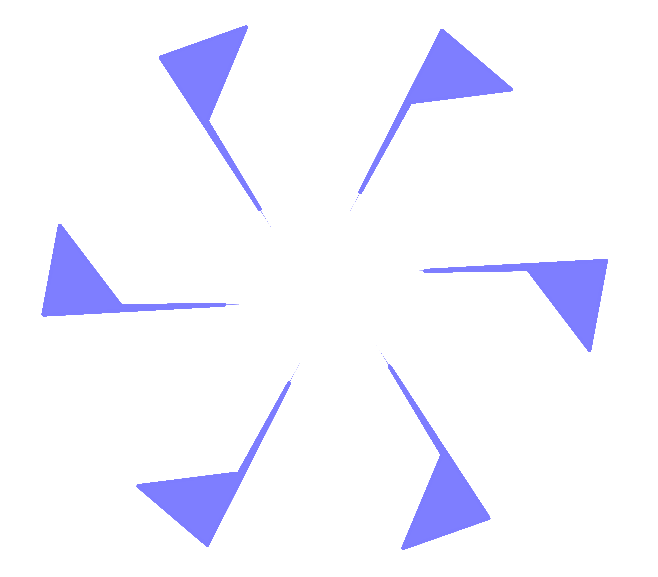#### You may also likeMake a set of numbers that use all the digits from 1 to 9, once and once only. Add them up. The result is divisible by 9. Add each of the digits in the new number. What is their sum? Now try some other possibilities for yourself!### N000ughty Thoughts

How many noughts are at the end of these giant numbers?### DOTS Division

Take any pair of two digit numbers x=ab and y=cd where, without loss of generality, ab > cd . Form two 4 digit numbers r=abcd and s=cdab and calculate: {r^2 - s^2} /{x^2 - y^2}.

# Robotic Rotations

### Why do this problem?

This problem appears at first to be about angles and rotations, but as students explore more deeply, they may be surprised to discover links to factors, multiples and primes. The images and interactivity provide an enticing hook to stimulate students' curiosity.

### Possible approach

Begin by showing students this image (PowerPoint Slide"Have a look at this image. What do you notice?"
Give students a short time to look at the image in silence, and then invite them to discuss with their neighbour.
"How do you think the image might have been created?"
Again, give students some thinking time and discussion time, and then share ideas as a class.

If computers are available, this would be a good time to introduce the interactivity.
"The image was created using this Rotation Robot. Work with a partner to explore the interactivity, and see if you can recreate the image."

As students make a start on the challenge, hand out this worksheet with eight more patterns for them to recreate.

While students are working, circulate and listen for pairs who are developing strategies or who have particularly useful insights. Once most students have successfully recreated most of the patterns, bring the class together and invite pairs to share their strategies and insights. Then draw students' attention to the last part of the task:

"I wonder if we can find a link between the angle and the number of copies of the image in the pattern... In a while, I am going to create a shape and choose an angle. I want you to be able to predict how many copies of the shape will appear."

Give students some more time to explore this using the interactivity. Then in the final plenary, you could challenge them with a few angles and ask them to predict and explain how many copies there will be:
"$160^{\circ}$?"
"There will be nine copies, because the highest common factor of 160 and 360 is 40, and 40 goes into 360 nine times."
"$305^{\circ}?$
"There will be 72 copies because the HCF of 305 and 360 is 5, and 5 goes into 360 72 times."

### Key questions

How did you work out which angle to rotate by?
Which patterns can be created using more than one angle?
Are there "families" of angles that make a particular rotational symmetry?
How can you categorise the families?
What is the link between the angle and the number of copies?

### Possible support

You may wish to try Attractive Rotations with your students before working on this problem.

### Possible extension

Challenge students to explain why the number of copies is related to the highest common factor of the angle of rotation and $360^{\circ}$. Stars would be a good problem to investigate alongside this.